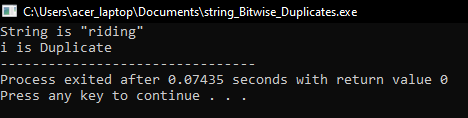# Finding Duplicates in a String using Bitwise Operations in C

## Finding Duplicates in a String using Bitwise Operations in C

In this article, I am going to discuss Finding Duplicates in a String using Bitwise Operations in C Language with Examples. Please read our previous article where we discussed How to Find Duplicates in a String in C Language with Examples.

##### How to Find Duplicates in a String using Bitwise Operations in C Language?

In our previous article, we discussed how to find duplicates in a string using HashTable. In this article, we will see how to find duplicates in a string using Bitwise Operations. We have already seen two methods for finding duplicate letters in a string. In this article, we will learn how we can perform the same thing using bits. This method is not just for strings, it is also useful for integer numbers also but it is more favourable for a string.

Let’s see this method. For learning this method, we should have some concepts here. Let us learn those concepts then we will find duplicate elements in a string.

##### Bitwise Operations:
1. Left Shift (<<)
2. Bits OR’ing (Merging)

We should know bitwise operations. We should know left shift and bits OR‘ing which is also called Merging and bits AND’ing which is also called Masking. We should know these things. Then we will see how to find duplicates. We will explain these operations one by one.

For understanding Bitwise operation, we should know how the data is stored in the memory in the form of bits. To understand that we have taken just one byte so it is sufficient to understand by just using one byte.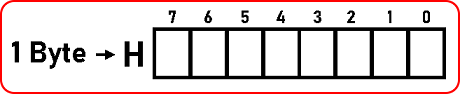We have a variable that is taking only one byte to let a character type variable. So, we’re calling that variable ‘H’ and it is taking only one byte so 1 byte is equal to 8 bits.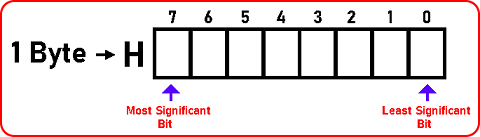This is indexed from 0 to 7 starting from the right-hand side. We started from the right-hand side so 0 is the least significant bit and 7 is the most significant. Now how any number is stored in the form of binary 0 and 1. For this, we should know the binary number system. Now we will understand Bitwise operations so for that let us assume that ‘H’ is initially 0.

Char H = 0;

If I declare a variable of type character and h assigned to 0 then zero will be stored. But how 0 will be stored: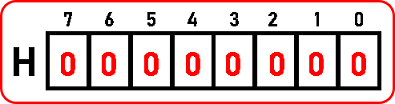All these will be zeros then if suppose we store 1 then: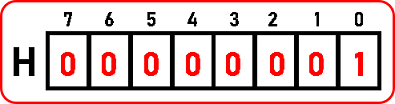So how it look like in the memory. We usually read from the left-hand side. Then if we store 2 here:

Char H = 2;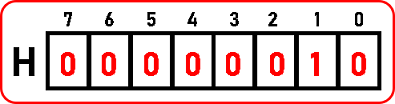The format is 2’s exponent is incremented from right to left side: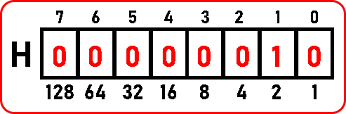Below are the binary forms for 1 to 10 numbers: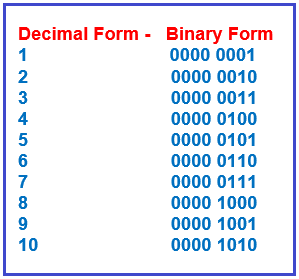So, if suppose we want to store 10, then 8th and 2nd will be ‘1’ and all are ‘0’. Suppose we want to store 20. The 16th and 4th will be ‘1’ and all will be ‘0’. So, we have seen how the binary form of a number is stored in the memory.

##### Shift Operation:

Next, we will see what it means by the shift operation.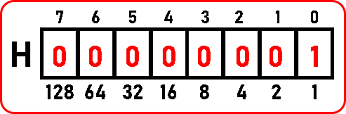Here we have ‘1’ stored in binary form as seen in the above image. What is meant by H << 1? We want to perform left shift operation in ‘H’ by ‘1’. So, whatever the value of ‘H’ is or whatever the bits are ‘1’, all the bits will shift by one place on the left-hand side. Then ‘H’ becomes: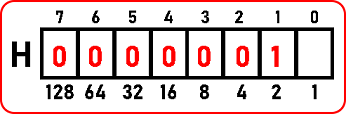So, all the bits have moved to one place on the left-hand side.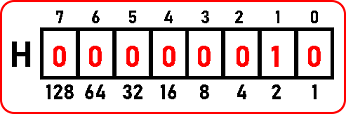And all the vacant places will be filled by zero. If the bits are shifting on any side, then we get some blank spaces. So that will be set as zeroes. But now what is this number. It has become too. Then let us put it back as:Not if we left shift by two places i.e. H << 2. Here all the bits will be shifted to 2 places on the left-hand side as follows: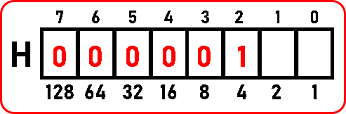And again, all the vacant places will be filled with zeros as: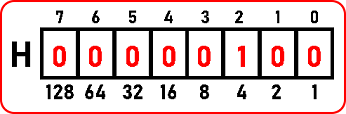This ‘H’ will modify and it will become 4. It means by shifting the number on the left-hand side we are able to increase it multiples by two and also that digit is shifting. Suppose here we have left-shifted by 5: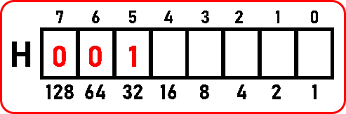And all the vacant places will be filled with ‘0’: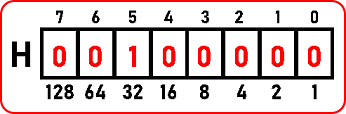Now it has become 32 here. In the same manner, we can perform the right shifting which will happen in the right direction i.e. H >> 5. So, we have explained to you shifting now we will explain to you AND’ing.

##### AND’ing: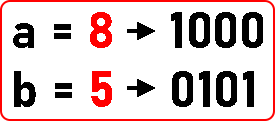For explanation, we have taken an example of two variables. We have taken just four digits binary form of those numbers because the rest of the bits on the left side are zero. Now, what is AND, If we say a & b then bits will ANDED?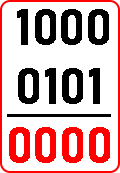There are some rules which we have to follow in AND operation: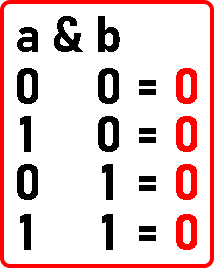There is only one condition when we will get 1. And this will only be possible when both values will be 1 only otherwise, we will get 0. Remember one thing we have used bitwise ‘&’ not logical and operator ‘&&’. So, from the above example where a = 8 and b = 5, the result of a & b is 0. Just remember the rules and apply them to other digits. So, in this manner, we have learnt AND’ing. Let us see OR’ing:

##### OR’ing:

For explaining OR’ing, we are taking the same example that we used in AND’ing:What does mean by OR’ing? If we replace the ‘&’ operator with ‘|’ between a and b like ‘a | b’ then it will be known as OR’ing. Let’s perform OR’ing in those bits: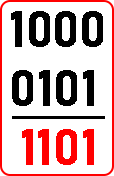There are some rules which we have to follow in OR operation: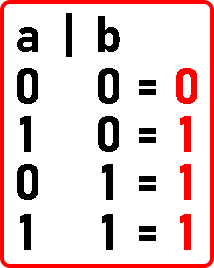There is only one condition when we will get 0. And this will only be possible when both values will be 0 only otherwise, we will get 1. Remember one thing we have used bitwise ‘|’ not logical and operator ‘||’. So, from the above example where a = 8 and b = 5, the result of a | b is 13.

Just remember the rules and apply them to other digits. So, in this manner, we have learnt OR’ing. Now let us understand what it means by merging and masking. Let us understand masking first.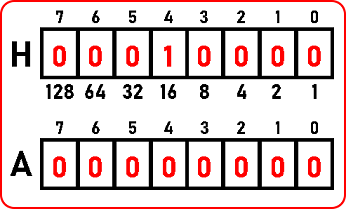For explaining masking, we have a variable ‘H’ of 1-byte size and the value in that one is 16. Then we have another variable ‘A’ in which everything is ‘0’. Now we want to know whether inside ‘H’, any bit is on or not means is it 1 or 0. Let’s take the example of the 2nd bit which is 0. But we want to find is it 0 or 1. So, we will take the help of ‘A’. Here we assign A = 1: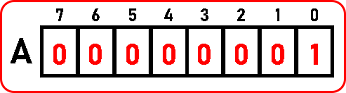Now, we want to know 2nd bit in ‘H’. So, perform left shift operation in ‘A’. A << 2; So,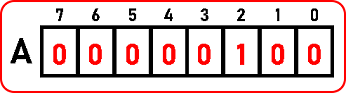We left-shifted ‘A’ by two places. Now the value of ‘A’ is 4 in decimal form. And the value of ‘H’ is 16 in decimal form. How do we know whether 2nd bit is on or not in ‘H’? We will perform AND’ing here.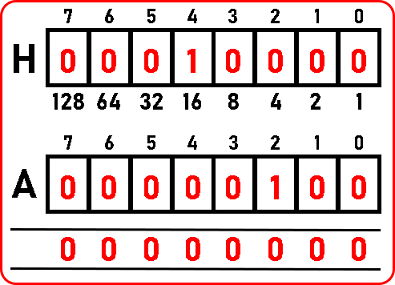The result of A & H is zero. As we got all the bits are ‘0’. It means 2nd bit is not on or ‘1’ in ‘H’. If we got a non-zero value means the bit is on or ‘1’. By performing and between ‘A’ and ’H’, we can know whether that bit is on or not.

So, knowing a particular bit inside of memory whether it’s on and off is known as masking. We have checked for only 2nd bit. You can check for other bits also. Now next we will see what is merging.

##### Merging:Here 4th bit is already on in ‘H’. We want to set 2nd bit as on Inside ‘H’. On the 2nd bit of ‘H’, we will take the help of ‘A’. First, initialize ‘A’ by 1 and left shift by 2 as:

Char A = 1;

A << 2;Now we will perform OR between ‘A’ and ‘H’ and store that result in ‘H’ as:

H = A | H;

Now we know the result of 0 and 1 will be 1. So here when we perform OR between ‘H’ and ‘A’ or 2nd bit of ‘H’ and 2nd bit of ‘A’ then it will result in 1. After performing OR’ing, we are storing the result in ‘H’ itself. So, this will on the 2nd bit of ‘H’ or set it to 1. So, the result of A & H is 0001 0100. So already some bits will be on and we have set the 2nd bit on that is called merging.

Checking whether a bit is on or off is known as masking. So, these two operations we have seen in Bitwise operations: left shift, masking and merging. All these operations we will use now for finding duplicates in a string.

##### Find Duplicates in a String using Bitwise Operations in C Language:

We will use masking and merging to find out are there any duplicates in a string.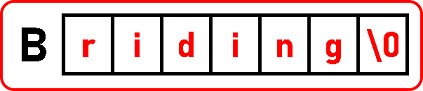We have taken an example sting where ‘i’ is repeating. So just we can find out are there any duplicates or not. We cannot count how many times that element or a character is repeated by using bits. So just we’ll find out whether the bits are already there or not. This procedure is similar to hashing.

We need some space. In hashing, we have taken an array of size 26. Now we need 26 bits but cannot get 26 bits we get in terms of bytes. So, 8 bits make 1 byte so we can get 32 but that is larger than 26. Otherwise, we will get 16 bits which is smaller than 26.

We know that a long integer takes 4 bytes. We are assuming that the integer takes 2 bytes. So, if int takes 2 bytes then long takes 4 bytes. But in some compilers of C / C++ integer itself takes 4 bytes. So just, in that case, int is sufficient you don’t have to pick long for it.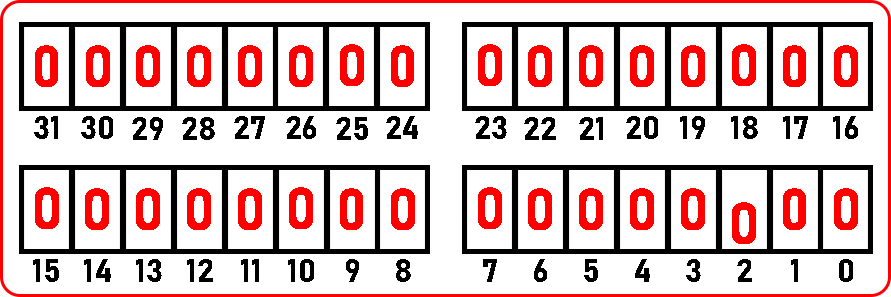So, 0 is the least significant bite. And 31 is the most significant bite. Now let’s see the code part:

##### Program to Find Duplicates in a String using Bitwise Operations in C Language:
#include <stdio.h>
#include <stdlib.h>
int main ()
{
char B[] = “riding”;
int H = 0, x = 0;
printf (“String is \”%s\”\n”, B);
for (int i = 0; B[i] != ‘\0’; i++)
{
x = 1;
x = x << (B[i]97);
if ((x & H) > 0)
{
printf (“%c is Duplicate”, B[i]);
}
else
H = x | H;
}
return 0;
}

Output: